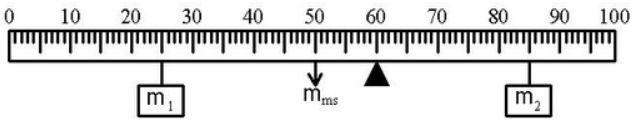# Problem: In the figure: the mass m1 is 0.70 kg and it is located at x1 = 25 cm.The pivot point is represented by the solid triangle located at x = 60 cm. The center of mass of the meter stick (mms = 0.40 kg) is located at its geometric center, xms = 50 cm. The mass m2 is 0.34 kg and it is located at x2 = 85 cm. (a) Calculate the net torque about the pivot (in N·m with the proper sign) due to these three weights. Use g = 9.79 m/s2.(b) True/False -- The apparatus described in the previous question is in equilibrium.

###### FREE Expert Solution

(a) Calculate net torque about pivot (make sure to convert cm → m)

τnet = τ1 + τms + τ2

= m1 g r1 + mms g rms + m2 g r2

= g( m1 (0.60 - x1) + mms (0.60 - xms) + m2 (0.60 - x2) )

(Subtracting each x from pivot means CCW torques are + and CW torques are −)

88% (319 ratings)###### Problem Details

In the figure:

• the mass m1 is 0.70 kg and it is located at x1 = 25 cm.
• The pivot point is represented by the solid triangle located at x = 60 cm.
• The center of mass of the meter stick (mms = 0.40 kg) is located at its geometric center, xms = 50 cm.
• The mass m2 is 0.34 kg and it is located at x2 = 85 cm.

(a) Calculate the net torque about the pivot (in N·m with the proper sign) due to these three weights. Use g = 9.79 m/s2.(b) True/False -- The apparatus described in the previous question is in equilibrium.